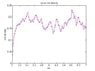# Lattice-gas Monte Carlo Simulation

gavincraig
Hi all...

Im trying to model a simple lattice-gas ( ising model ) system , using MC metropolis algorithm. I am having trouble with the code. I am trying to do it in matlab. My lattice gas does not show the characteristics it should. I used my old Ising Model code, which I modified to do particle exhange, to keep the number of particles constant, too see the effects in the canonical ensemble, not the grand canonical ensemble, as is the case with "normal" ising model spin-flip
metropolis algorith...

I need someone to spot the error, that for weeks I was unable to see. This is a little side project...

Code:
unction sorption7

L = 20;             %Lattice Size
N = 1000;           %MC steps

epsilon = 1;        %Interaction Term
J = epsilon;

%k = 1;
k = 1.3806503 * 10^(-23);       %Boltzman constant

MU = linspace(5,7,100);         %chemical potential
mu2 = 7;                       %chemical potential in secondary region

T0 =0.5;                        %Temp
T_reduced = (k*T0)/epsilon;     %Reduced Temp
T = T_reduced;

beta = 1/(k*T);                 %beta

%p = rand(L);
%p( find(p < 0.5) ) = -1;
%p( find(p > 0.5) ) = 1

p = zeros(L);

p(:,:) = -1;
p(1:8,1:8) = 1
QM = zeros(1,length(MU));

cnt = 1;
while cnt < length(MU)+1,

mu  = MU(cnt);
mecnt =  0;
mctime = 0;
aveMQ = 0;
while( mctime < N ),
mecnt = mecnt + 1;
Q = length(find(p(:,L/2:L)==1));
aveMQ = aveMQ + Q/(L*(L/2));

gop =find(p==1);
gop_L = length(gop);

jut = round(1+ (gop_L-1)*rand(1));

[i,j] = ind2sub(size(p),gop(jut));
idx = move( i,j, p,L/2 );
%current_index = sub2ind( size(p), i,j);
current_index = gop(jut);
if( idx ~= current_index && idx ~= 0 ),
[de ep] = delta6( J, mu,mu2,idx,p,10);
%[de ep] = delta6( J, mu,mu2, current_index,p );
%de = de -1;
%de = ((de2-1)-de1);
%ep = (ep2-ep1);
%aveEP = aveEP + ep;
if de <= 0,
p(idx) = 1;
p(current_index) = -1;
end;
if de > 0,
rr = rand(1);
if rr < exp( -de*beta ),
p(idx) = 1;
p(current_index) = -1;

end;

end;

end;

if idx == 0,

end;

NN = length( find(p==1));
ss = sprintf(' Number of Particles %d',NN);
image(p+1);
title(ss);
colormap(hsv(4));
drawnow;
mctime = mctime + 1;
end; %while MC LOOP

QM(cnt) = aveMQ/mecnt;
cnt = cnt + 1;
end;
figure;
plot(MU,QM,'.-m');
title('mu vs site density');
xlabel('mu');
ylabel('site density');
end

This is my hamiltonian, that I derived from the Ising Model hamiltonian/Energy for external magnetic field...

Code:
function E = Hamiltonian( mu, J, Ep, N_s,N_o )
mu0 = 4*J;
mu1 = 2*mu - 8*J;
term1 = N_s*(mu-2*J);

E = -mu0*Ep - mu1*N_s; + term1;
end

What I am trying to achieve is the simple phase transition. in my llittle model I have 2 regions. 1 region is kept at a constant chemical potential, while the other region I start from a low chemical potential moving up. I am expecting there to be some kind of phase transtion, where the particles will favour, mostly, but not all, 1 region above the other. What I see is in the graph attached. There is periodic BC in all directions.

My question is thus... is the code modelling it correctly? and is the phase transition I see due to the BC... I've altered my BC so that it has only say left/right periodicity, and not up/down, and it produces the same type of density plot...

thanks

#### Attachments

•untitled.jpg
16.6 KB · Views: 400